# Going to a Movie

Mr. Murphy is taking some students to see a movie. Mr. Murphy needs a seating arrangement for thirty students. Mr. Murphy wants an equal amount of students in each row. What are all the possible seating arrangements Mr. Murphy can make? Show all your mathematical thinking.

Assessment

## Plan

#### Formal Mathematical Language and Symbolic Notation

A student may independently select a printed number line, number chart, ten frames, graph paper, etc. as they work on a task.

### Suggested materials

Engagement Image:

Teachers may project the image below to launch this task for their students, define nouns, promote discussion, access prior knowledge, and inspire engagement and problem solving.

## Solutions tabs

### Expert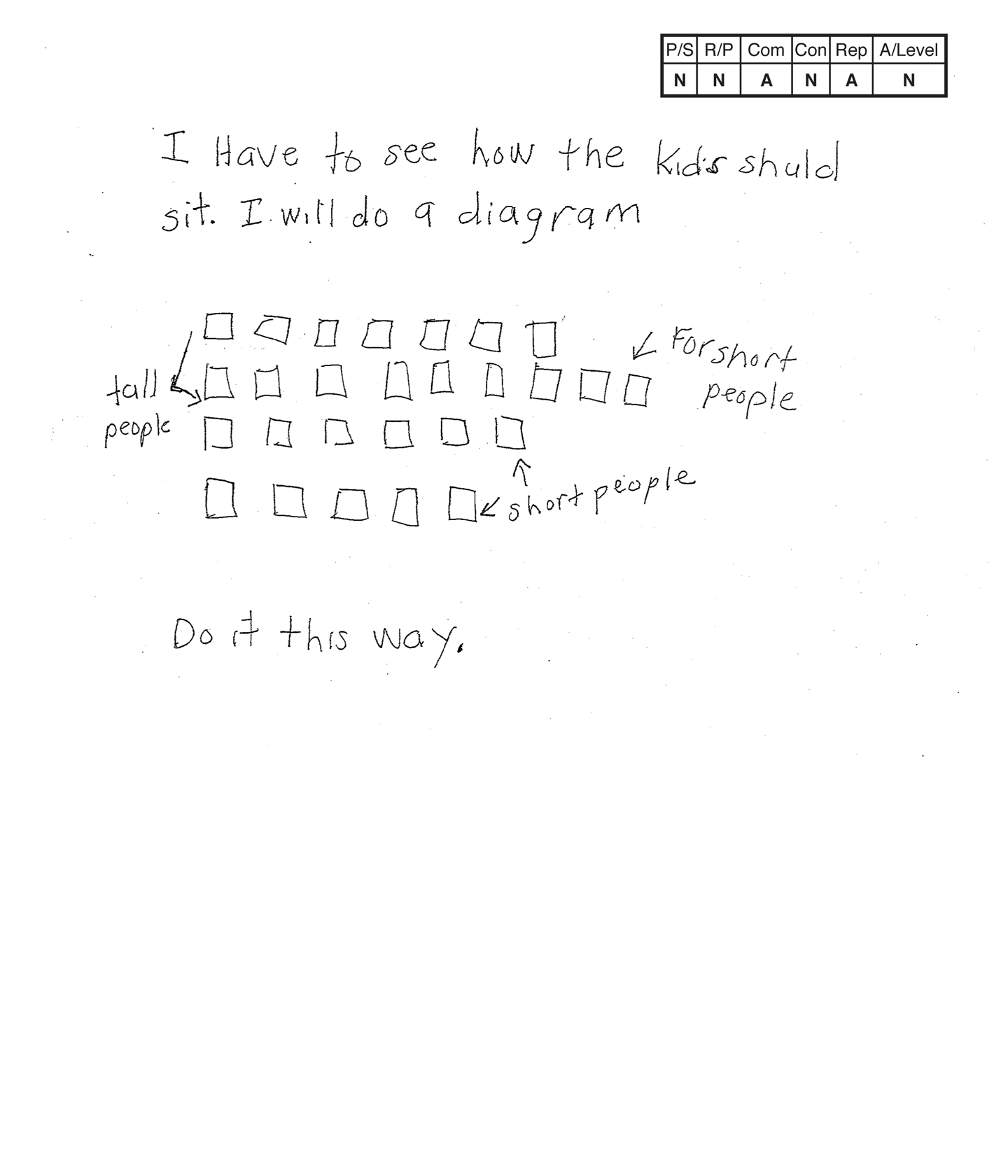This student does not meet the standard.

### Scoring Rationale

#### Novice

The student's strategy of diagramming seats in four unequal rows and stating which seats would suit students of various heights would not lead to a correct solution. The student's answer, "Do it this way," is incorrect.

#### Novice

No correct reasoning of the underlying mathematical concepts of using multiplication and division within 100 involving equal groups, arrays, and measurement quantities is evident. The student does not demonstrate any understanding of arrangements of equal groups, arrays, or factors of 30.

#### Apprentice

The student correctly uses the mathematical term diagram and indicates understanding of the term but not the concepts involved in the task.

#### Novice

The student does not make a mathematically relevant observation about their solution.

#### Apprentice

The student's diagram represents seats but the purpose of the seats representing students of various heights is not appropriate to the task.

#### Novice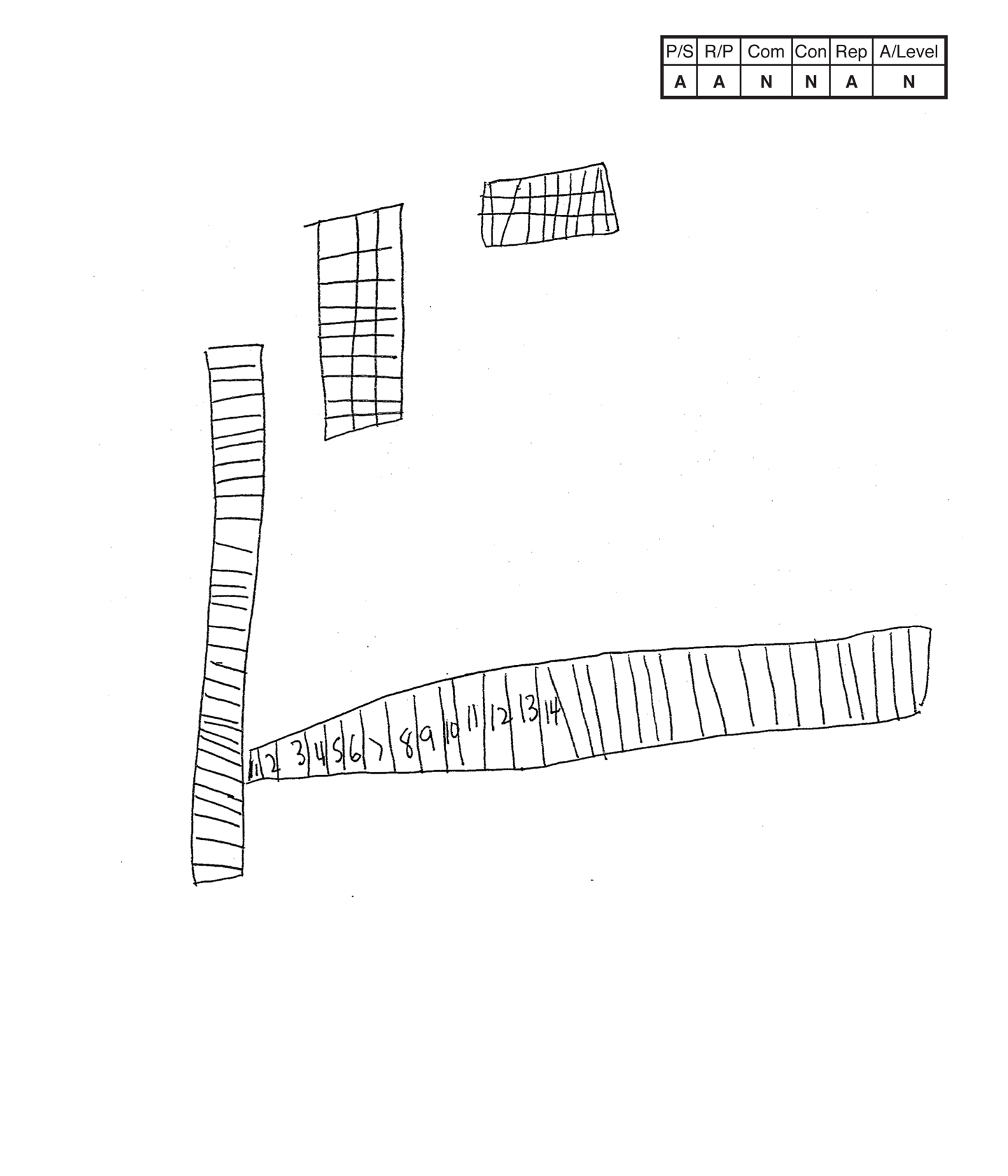This student does not meet the standard.

### Scoring Rationale

#### Apprentice

The student's strategy of using diagrams to show arrangements/arrays of the student seating would work to solve the problem. The student only correctly diagrams a 3 x 10 and 10 x 3 arrangement so they arrive at a partially correct answer.

#### Apprentice

The student has some correct reasoning of the underlying mathematical concepts of using multiplication and division within 100 involving equal groups, arrays, and measurement quantities. It is unclear why the student makes a 1 x 32 and a 32 x 1 arrangement.

#### Novice

The student does not use any mathematical language or notation. Scribing for this student may have led to use of mathematical language.

#### Novice

The student does not make a mathematically relevant observation about their solution.

#### Apprentice

The student attempts a diagram but only two arrangements/arrays are appropriate. No key or text is provided to support the arrangements/arrays.

#### Novice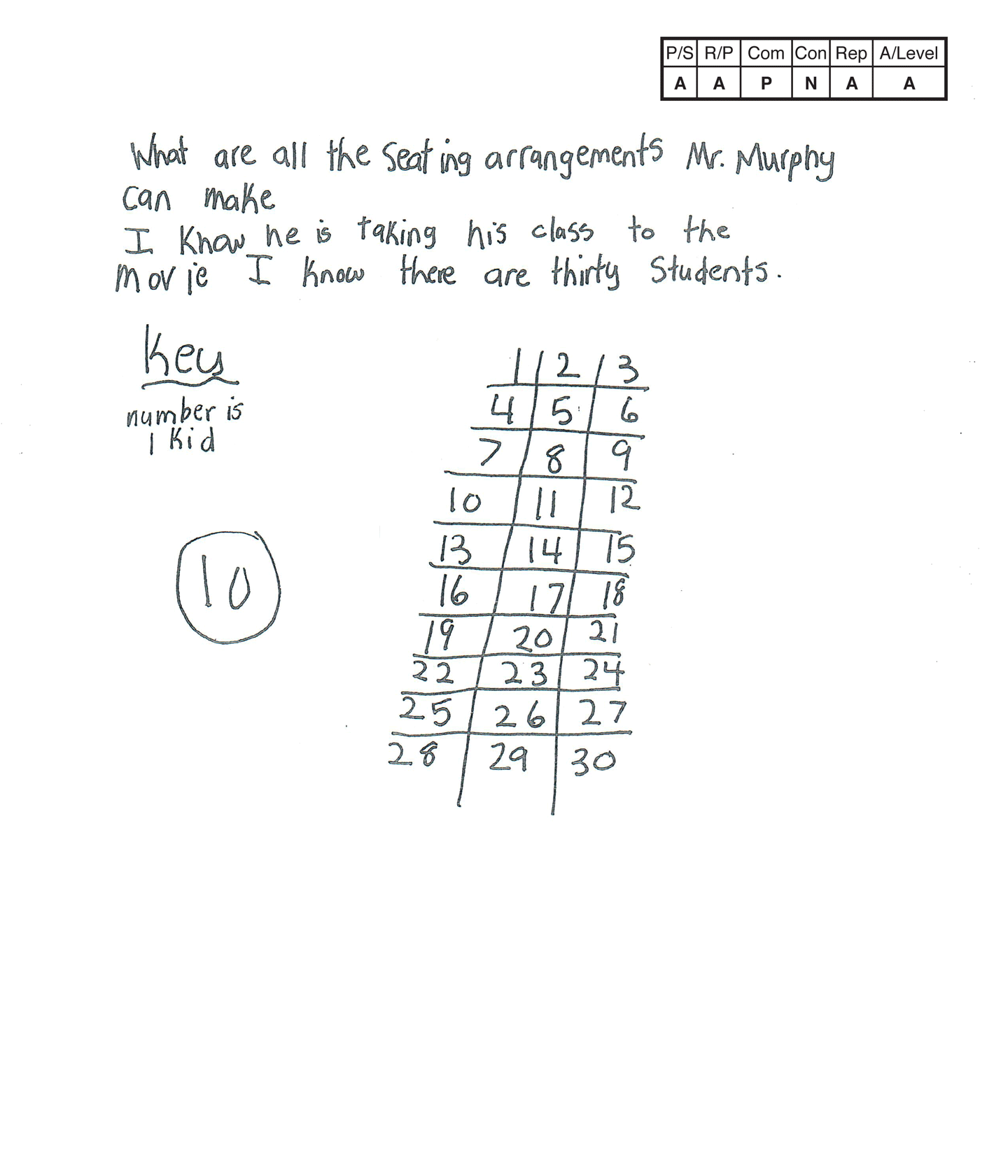,This student does not meet the standard.

### Scoring Rationale

#### Apprentice

The student's strategy of making a diagram and key of one possible seating arrangement for 30 students and then using grid paper to diagram that arrangement and five others would work to solve the task. The student does not diagram all the possible arrangements. The 2 x 15 and 15 x 2 arrangements are missing.

#### Apprentice

The student's solution contains some understanding of the underlying mathematical concepts of equal groups/arrays, but there are gaps in how the student solved the task and the reasoning used. It is not clear why the student draws six arrays and states an answer of ten. Two arrangements/arrays are labeled 1 x 2, which is not consistent with the others.

#### Practitioner

The student correctly uses the mathematical term arrangement from the task. The student also correctly uses the mathematical term key.

#### Novice

The student does not make a mathematically relevant observation about their solution.

#### Apprentice

The student's use of two different types of diagrams to show arrangements of student seating is appropriate to this task. The student's first diagram is accurate for one possible arrangement. There is a key to define the "kids" seating in each row and column. The student's arrangements/arrays on the grid paper all lack labels or a key to indicate what the arrays are indicating. The two 1 x 2 diagrams should read 1 x 30 and 30 x 1.

#### Apprentice

Note:

The overall achievement level for this piece of student work falls under Exemplars exception to the rule category. If a student has all Apprentice scores or above, but a Novice in "Connections," the student may still receive an achievement level score of Apprentice. To learn more about Exemplars scoring, please refer to the section of your dashboard called "Tools for Success" and click on the link for “Using the Assessment Rubric.”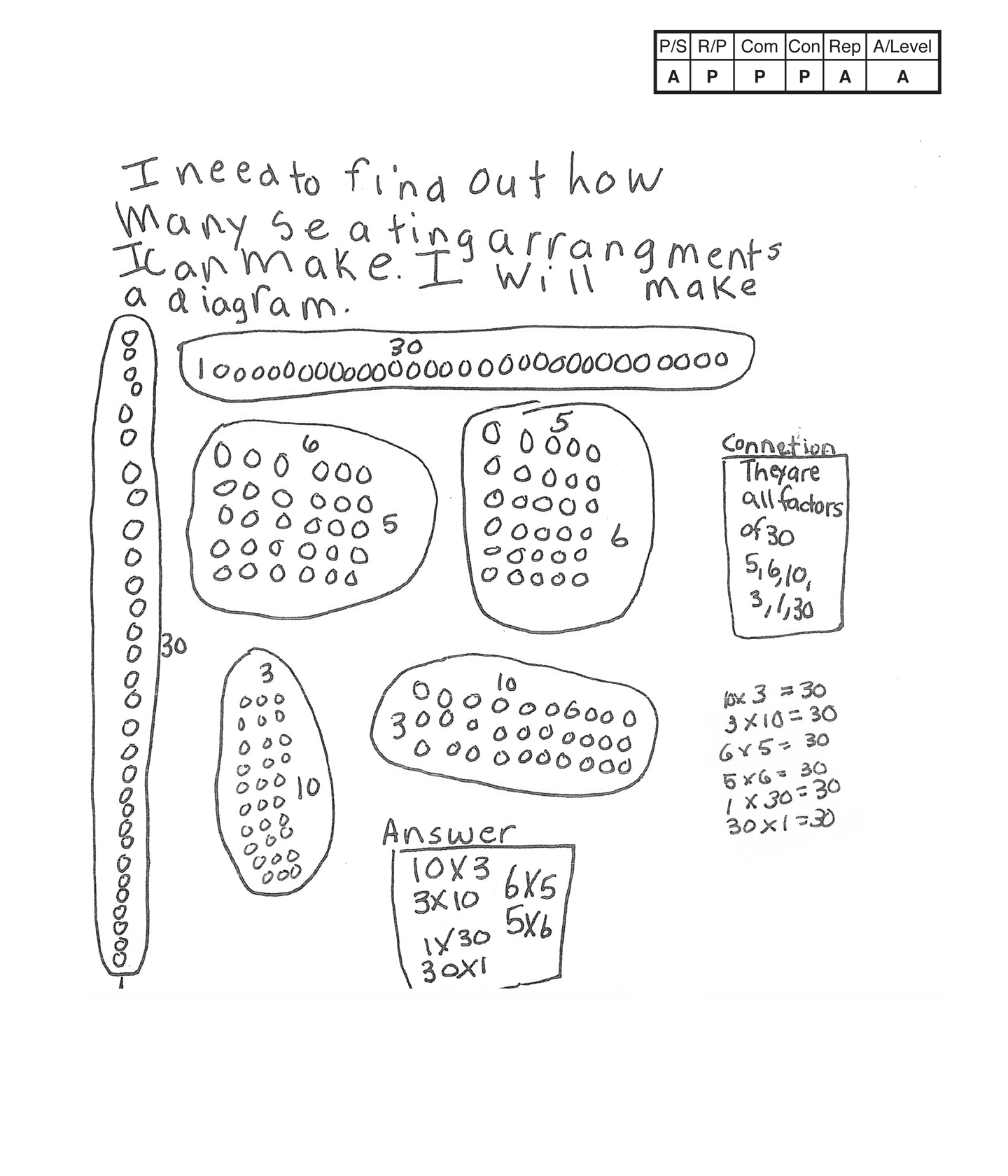This student does not meet the standard.

### Scoring Rationale

#### Apprentice

The student's strategy of using a diagram to make arrangements of how to seat 30 students would work to solve the task. The student's solution is correct for only part of the task. The student only identifies six of the eight possible seating arrangements.

#### Practitioner

The student demonstrates correct reasoning of the underlying mathematical concepts of using multiplication and division within 100 involving equal groups, arrays, and measurement quantities that is necessary to solve the task.

#### Practitioner

The student correctly uses the mathematical term arrangement from the task. The student also correctly uses the terms diagram and factors. The student uses the mathematical notation 10 x 3 (10 by 3), 3 x 10, 6 x 5, 5 x 6, 1 x 30, 30 x 1.

#### Practitioner

The student makes a mathematically relevant observation about their solution, "They are all factors of 30—5, 6, 10, 3, 1, 30." The student does not need to list all the factors of 30.

#### Apprentice

The student's diagram is appropriate but not accurate. There is no key or text to indicate that the arrays represent student seats. The 2 x 15 and 15 x 2 arrays are missing.

#### Apprentice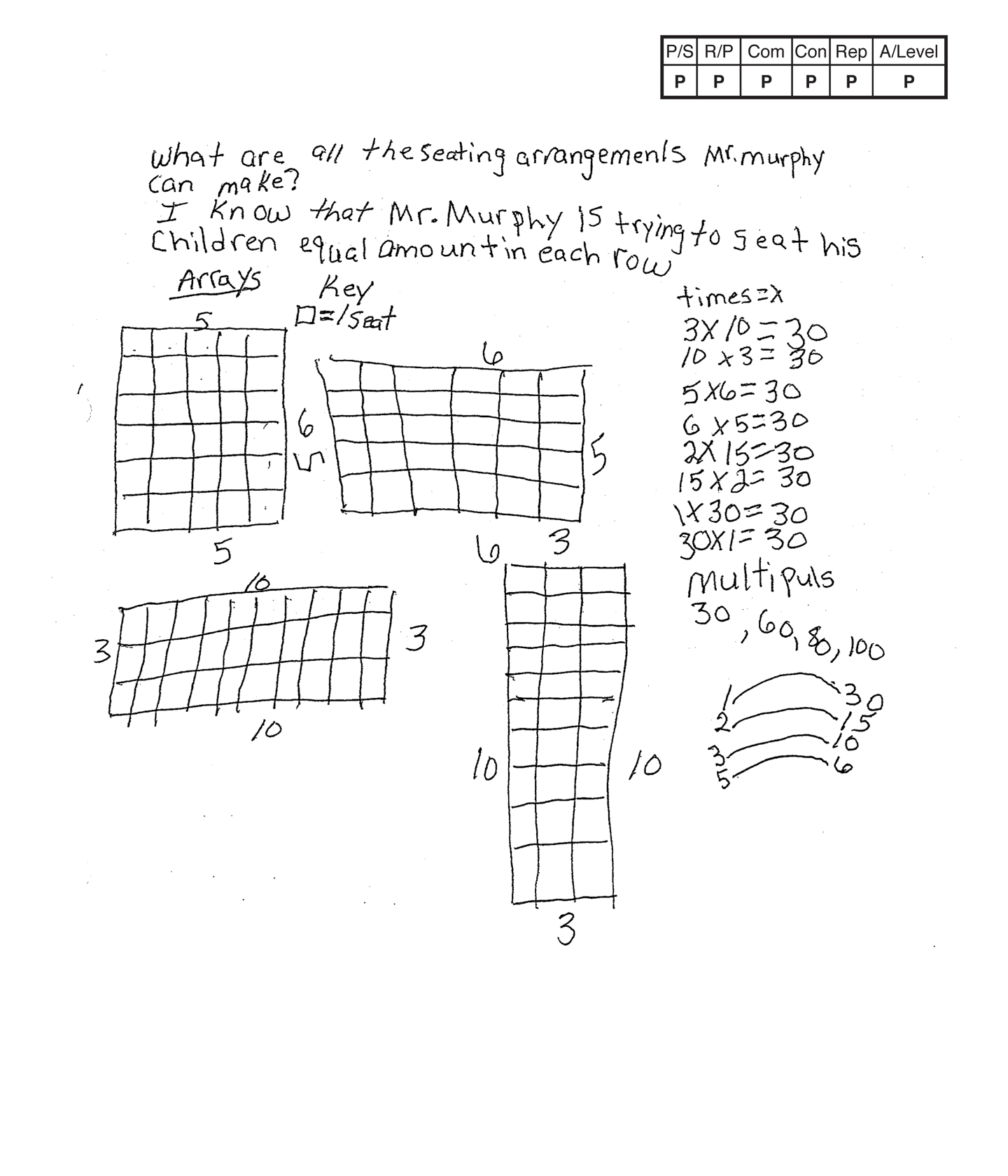,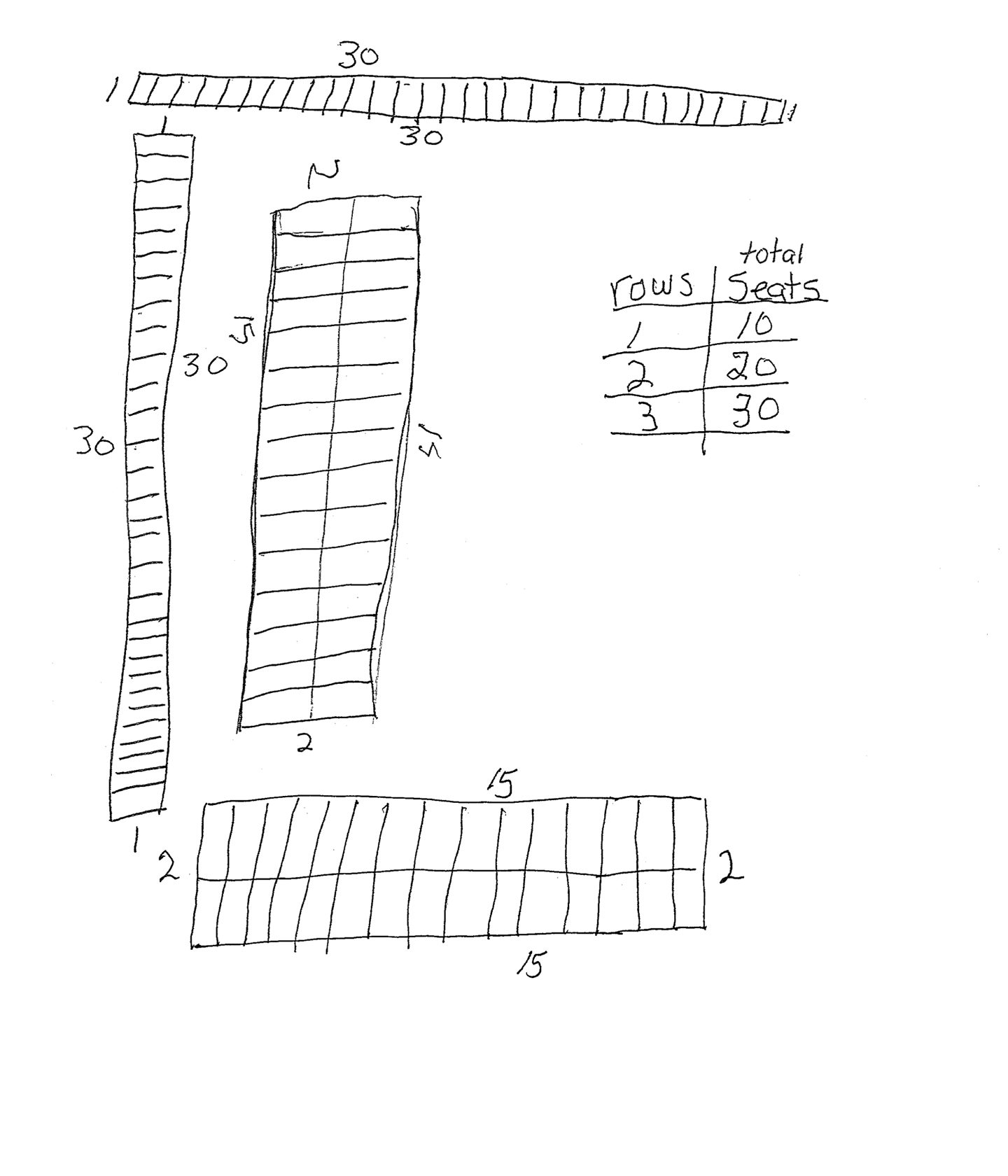This student meets the standard.

### Scoring Rationale

#### Practitioner

The student's strategy of making a diagram of arrays to find eight seating arrangements works for solving this task. The problem asks, "What are all the seating arrangements Mr. Murphy can make?" The student correctly diagrams eight arrays/arrangements and is not required to state 5 x, etc.

Note: Students can just indicate four possible arrangements for a correct answer as the understanding of Commutative property can be assumed.

#### Practitioner

The student demonstrates correct reasoning of the underlying mathematical concepts of arrangements/arrays.

#### Practitioner

The student correctly uses the mathematical terms arrangement, equal, amount, row from the task. The student also correctly uses the terms array and key. The student does not use the term "multipuls" (multiples) correctly so it cannot be considered as a mathematical term for this task.

#### Practitioner

The student connects their arrays to the factors of 30 in two ways. The student shows 1 with 30, 2 with 15, 3 with 10, and 5 with 6. The student also lists the multiplication notation with the reversals 3 x 10 = 30, 10 x 3 = 30…. The student does not earn credit for stating, "multipuls" 30, 60, 80, 100, because this is not correct.

#### Practitioner

The student's arrays are appropriate and accurate for this task. A key is provided to define what the arrangements/arrays are representing.

#### Practitioner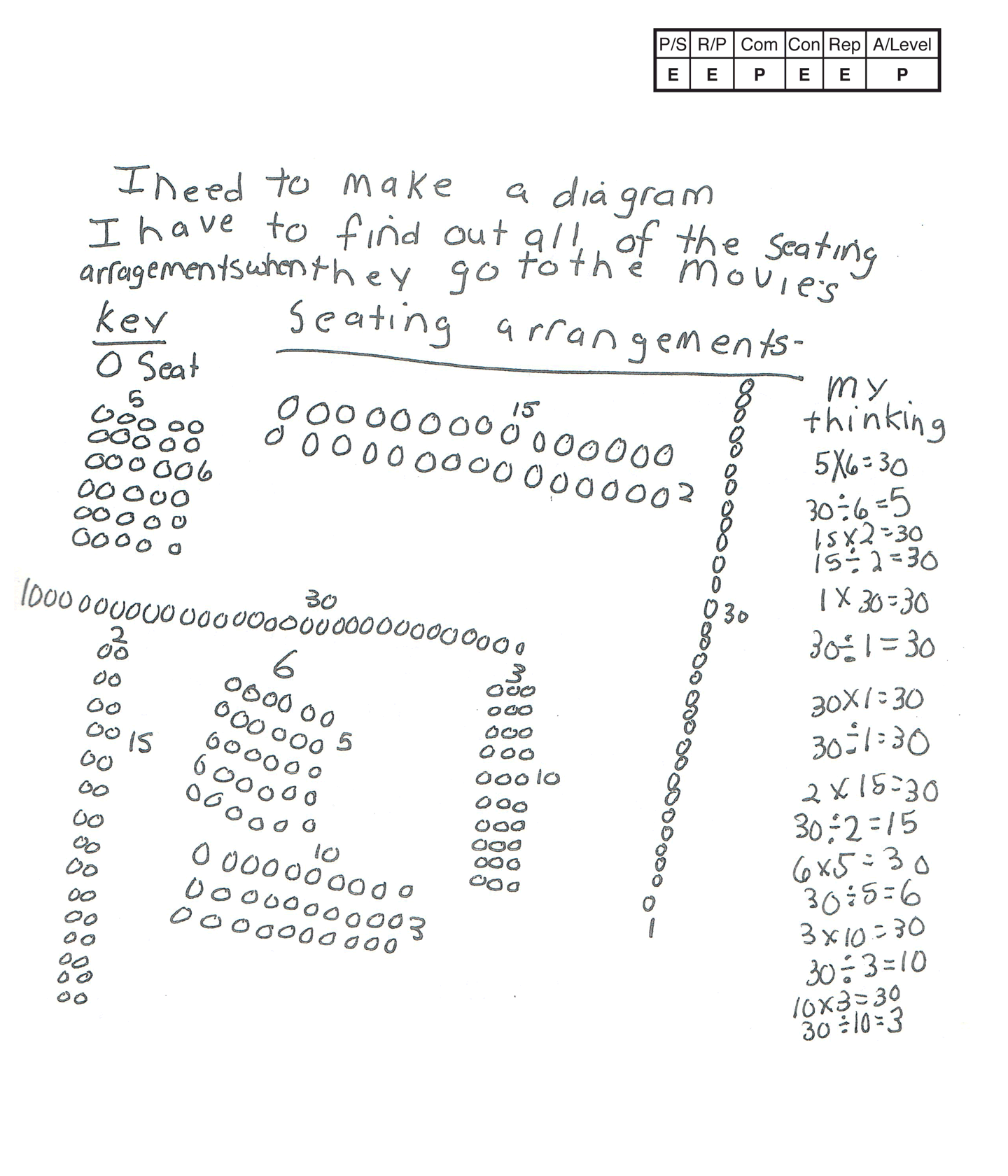,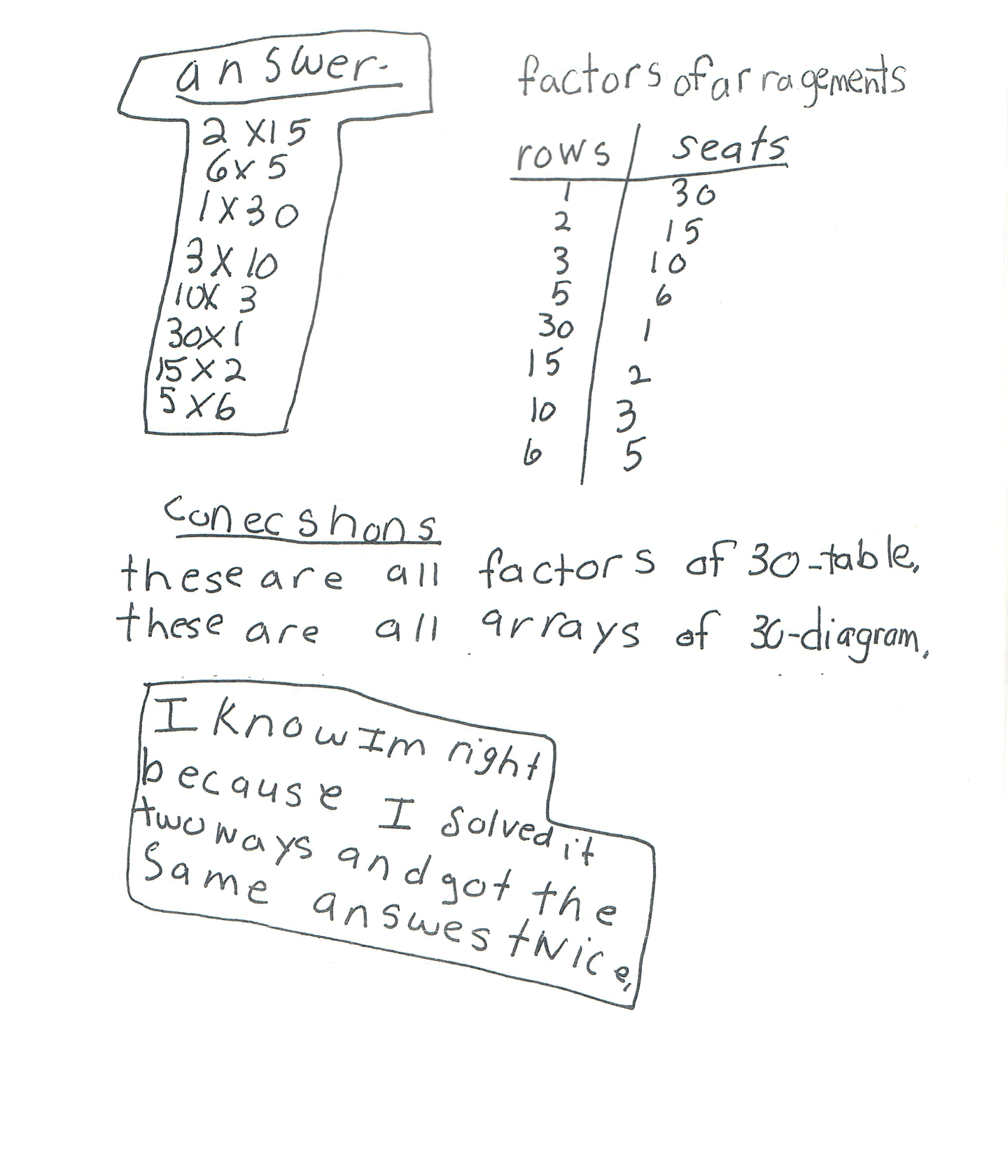This student meets the standard.

### Scoring Rationale

#### Expert

The student's strategy of making a table to find the eight possible arrangements/arrays for thirty people works for solving the task. The student's answer, "2 x 15, 6 x 5, 1 x 30, 3 x 10, 10 x 3, 30 x 1, 15 x 2, 5 x 6," is correct. The student verifies their answer by making a table of arrangements and linking the arrangements to factors.

#### Expert

The student demonstrates correct understanding of the underlying mathematical concept of arrangements/arrays that is necessary to solve this task and then uses the concept of factors and a table to verify that their answer is correct.

#### Practitioner

The student correctly uses the mathematical terms arrangement and row from the task. The student also correctly uses the terms diagram, key, factors, table. The student correctly uses the mathematical notation 2 x 15, 6 x 5, 1 x 30, 3 x 10, 10 x 3, 30 x 1, 15 x 2, 5 x 6.

#### Expert

The student first makes the Practitioner connections, "These are all factors of 30-table," and, "these are all arrays of 30-diagram." The student makes the Expert connection of verifying that their answer is correct. The student makes a table of "factors of arragements," and states, "I know Im right because I solved it two ways and got the same answes twice."

#### Expert

The student's diagram is appropriate and accurate for this task. A key defines the seats. The student's table is also appropriate and accurate with all necessary labels provided and all entered data is correct. The student compares the two diagrams to verify that their answer is correct.

#### Practitioner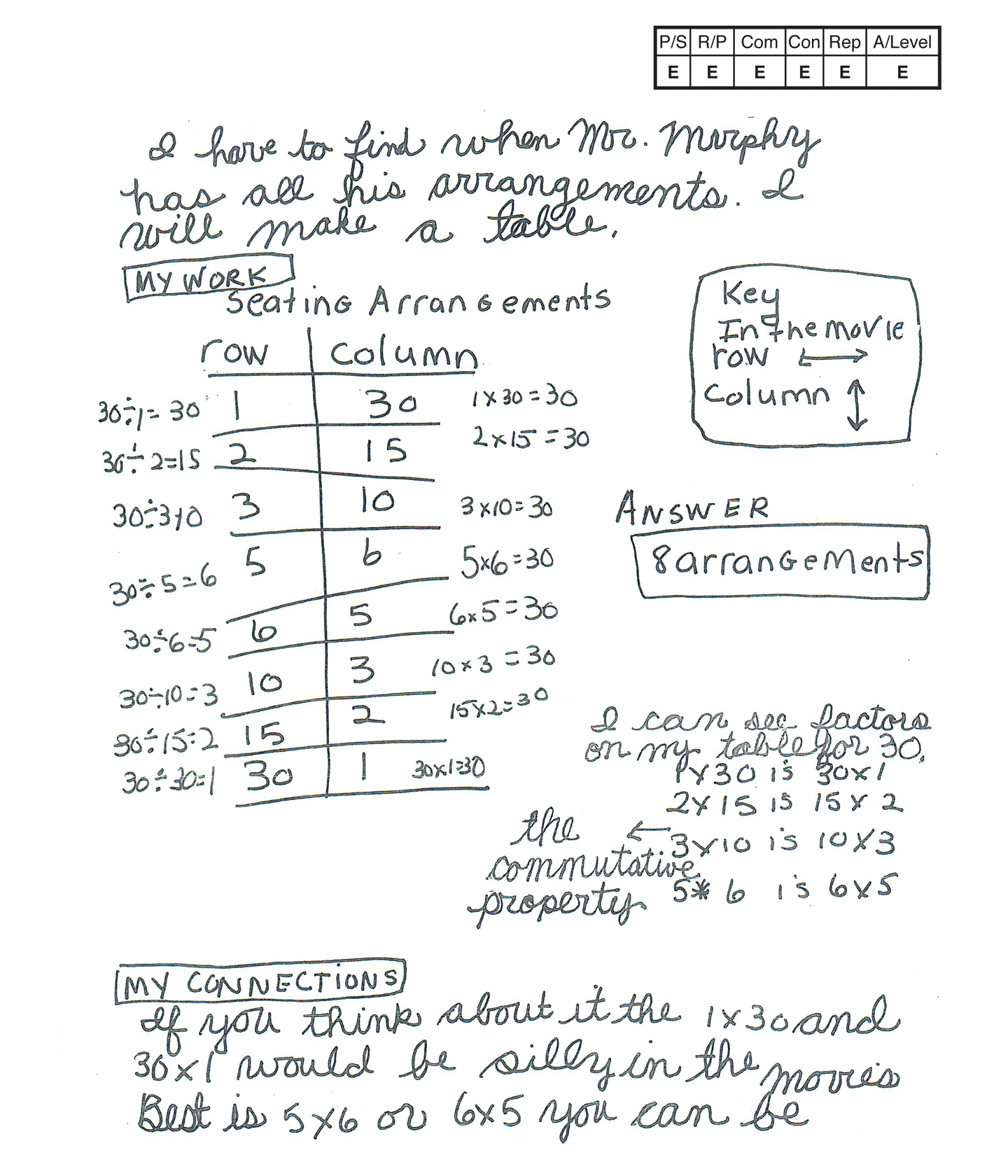,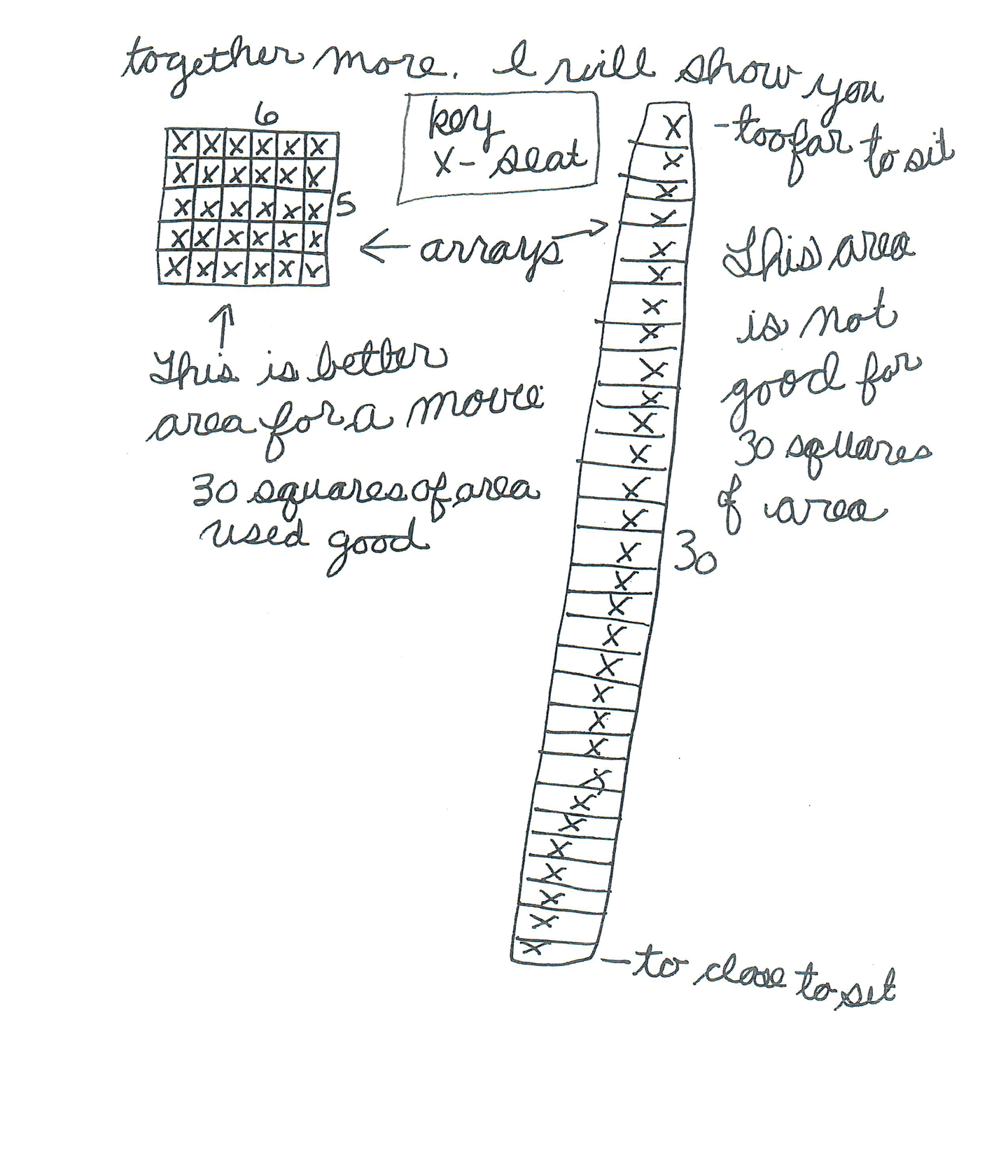This student exceeds the standard.

### Scoring Rationale

#### Expert

The student's strategy of making a table to find the eight possible arrangements/arrays for 30 people works for solving the task. The student's answer, "8 arrangements," is correct. The student also extends their work to the concept of area.

#### Expert

The student demonstrates correct understanding of the underlying mathematical concept of arrangements/arrays. The student brings correct reasoning of the the underlying concept of area to extend their solution.

#### Expert

The student correctly uses the mathematical terms arrangement and row from the task. The student also correctly uses the terms table, column, key, factors, commutative property, array, square and area. The student uses the mathematical notation 1 x 30 (1 by 30), 30 x 1, 2 x 15, 15 x 2, 3 x 10, 10 x 3, 5 x 6, 6 x 5.

#### Expert

The student states, "I can see factors on my table for 30," and lists them on their paper. The student then states, "the commutative property." The student analyzes the relationships among elements in their solution. The student states, "If you think about it the 1 x 30 and 30 x 1 would be silly in the movies. Best is 5 x 6 or 6 x 5, you can be together more. I will show you." The student uses arrangements/arrays to link the concept of area in a 6 x 5 arrangement/array as opposed to the area in a 1 x 30 arrangement/array. The student states, "This is better area for a movie. 30 squares of area used good. This area is not good for 30 squares." The student also defines the use of area and direction by indicating that the 1 x 30 would have seats "to far to sit" and "to close to sit."

#### Expert

The student's table is appropriate and accurate. All necessary labels are provided and the entered data is correct. The student's diagram is also appropriate and accurate to support their understanding of area.